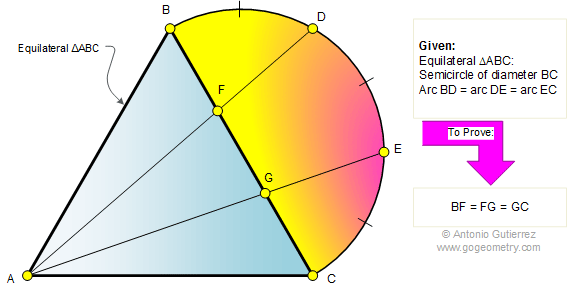Problem 326. Equilateral triangle, Semicircle, Equal arcs. Level: High School, SAT Prep, College geometry. The figure shows an equilateral triangle ABC. D and E are points dividing the semicircle of diameter BC into three equal arcs. Prove that the lines AD and AE divide the side BC into three equal parts.Recent Additions